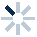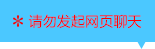| | |

||
APP端下载AndroidiPhone
|
￥42

|
15人点赞
93175人已学习
|

3天无理由退款

3天无理由退款：退款将以超级币形式退至您的超级课堂学习账户，便于您重新选购其他课程。恶意退款将被冻结账号。

• 1、对于五点法，设$X=\omega x＋\phi$，由$X$取$0$、$\frac{\pi }{2}$、$\pi$、$\frac{3\pi }{2}$、$2\pi$来求出相应的$x$值及对应的$y$值
2、 描点、连线就得到了一个周期内的图象
• 1、利用之前课程中介绍的三种变换，把$y=sinx$的图象转化为$y=Asin(\omega x+\phi )$的图象。从标准变复合，先相位变换更简单；从复合变标准，先周期变换更简单
2、 如果在这种复合函数的解析式再加上一个常数，$y=Asin(\omega x+\phi )+k$，就是再多了一个上下平移$\left | k \right |$个单位的步骤
• 1、主要介绍了三角复合函数图象的作法在习题中的应用
2、 同学们一定要熟悉图象变换
• 1、求$\omega$要通过周期，通常题目有两种给出周期的方式：一种是图象横向平移一段距离后会和自身重合。另一种是给出图象上特殊位置之间的距离
• 1、求$\phi$要通过代入图象上的点，在一个周期内，有四个特殊点方便代入：最高点、最低点，以及两个对称中心
2、 如果$A＜0$，那复合函数图象上的最高点、最低点、上升点、下降点，其$x$值就会分别对应中层三角函数的最低点、最高点、下降点、上升点
• 1、求$k$有两种方法。通过图象的平衡位置或值域
2、 求$A$也可以利用图象或值域，如果振幅、值域不清楚，或$A$的正负不确定，可以选择代入特殊点
3、 $A$、$k$、$y_{max}$、$y_{min}$这四个值之间，只要知道任意两个就能求出另外两个
• 1、主要内容是四种参数$A$、$\omega$、$\phi$、$k$在综合题中的应用
2、 同学们要对图象变换，字母意义，字母求法这些内容做到非常熟悉，它们是这个章节最常考、最常用的知识点
• 综合练习

• 1超级学员1464258
• 2超级学员
• 3超级学员1611235
• 4超级学员1725606
• 5超级学员2778458
• 6超级学员3613788
• 7超级学员3795261
• 8超级学员3
• 9超级学员3893843
• 10超级学员4129601196447人在学
￥ 78 ￥ 78127121人在学
￥ 78 ￥ 78219512人在学
￥ 0 ￥ 0126304人在学
￥ 72 ￥ 72

• 0
• 点击分享有好礼
••app端下载关注微信号